Research papers and code for "Sreeram Kannan":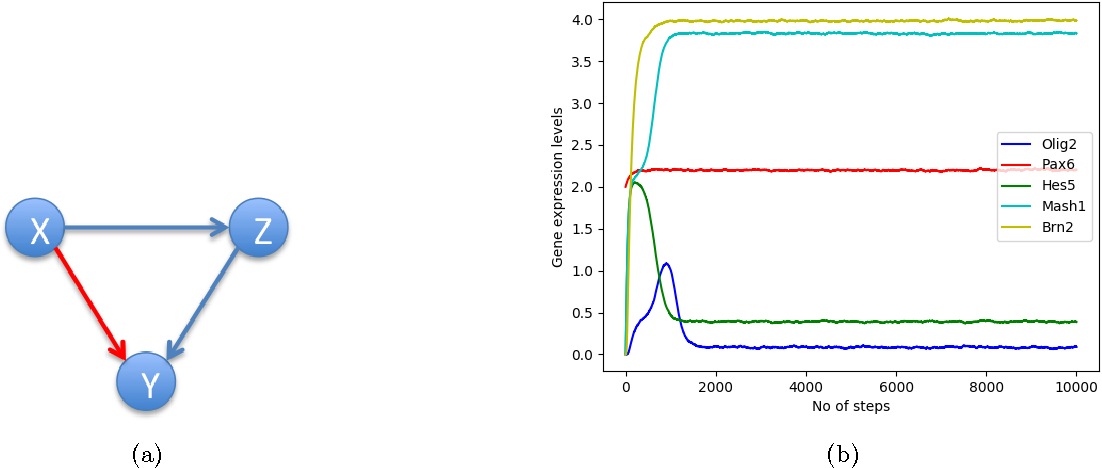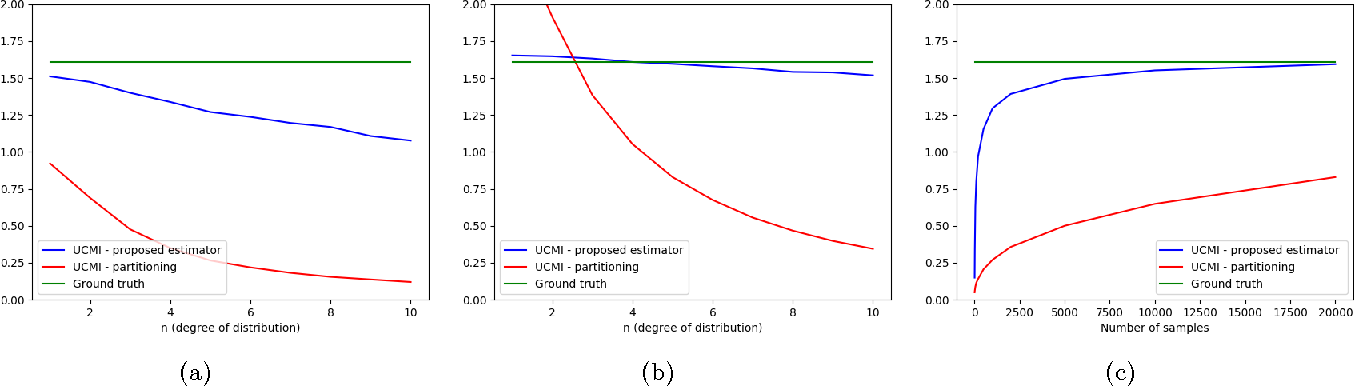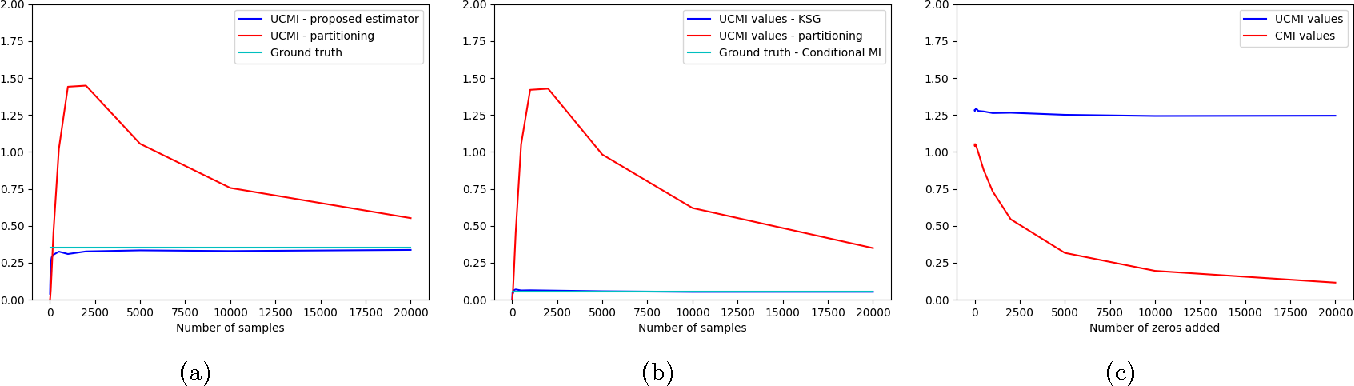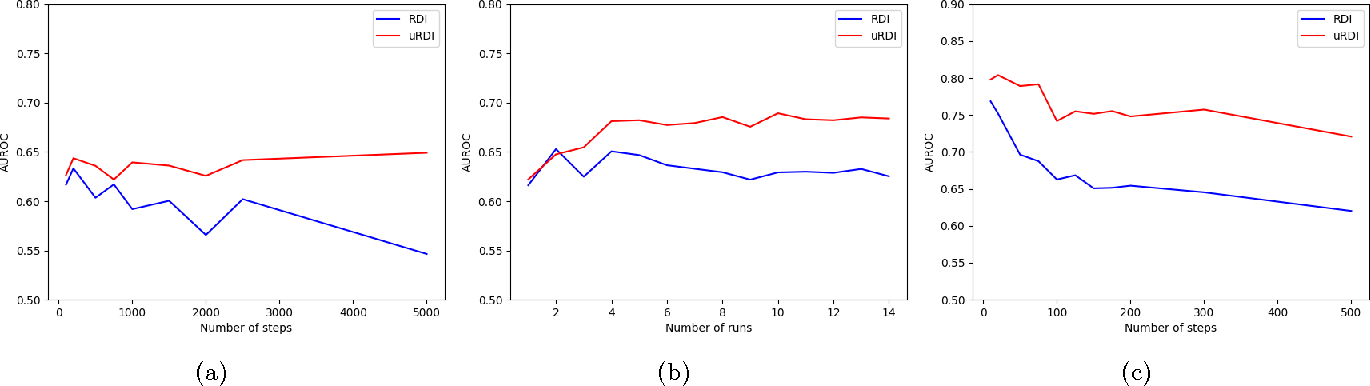The conditional mutual information I(X;Y|Z) measures the average information that X and Y contain about each other given Z. This is an important primitive in many learning problems including conditional independence testing, graphical model inference, causal strength estimation and time-series problems. In several applications, it is desirable to have a functional purely of the conditional distribution p_{Y|X,Z} rather than of the joint distribution p_{X,Y,Z}. We define the potential conditional mutual information as the conditional mutual information calculated with a modified joint distribution p_{Y|X,Z} q_{X,Z}, where q_{X,Z} is a potential distribution, fixed airport. We develop K nearest neighbor based estimators for this functional, employing importance sampling, and a coupling trick, and prove the finite k consistency of such an estimator. We demonstrate that the estimator has excellent practical performance and show an application in dynamical system inference.

Click to Read Paper and Get Code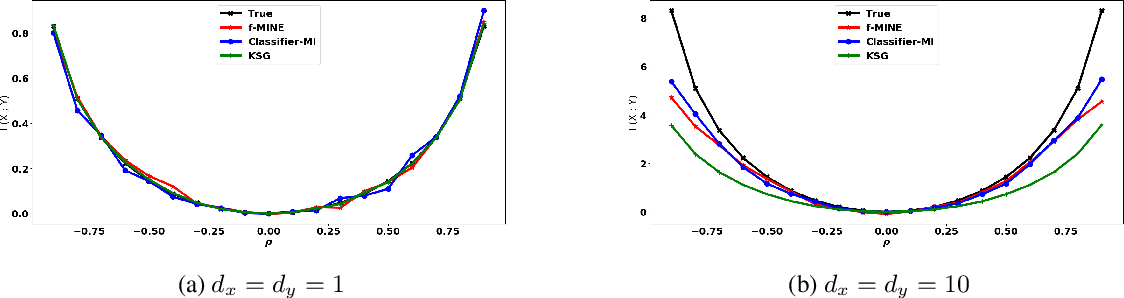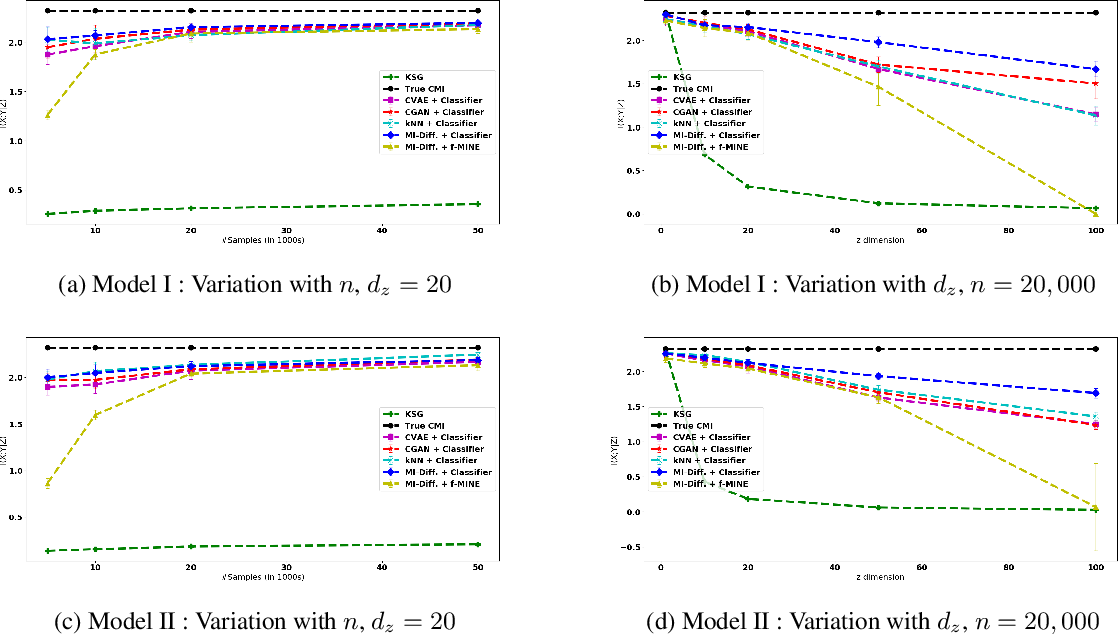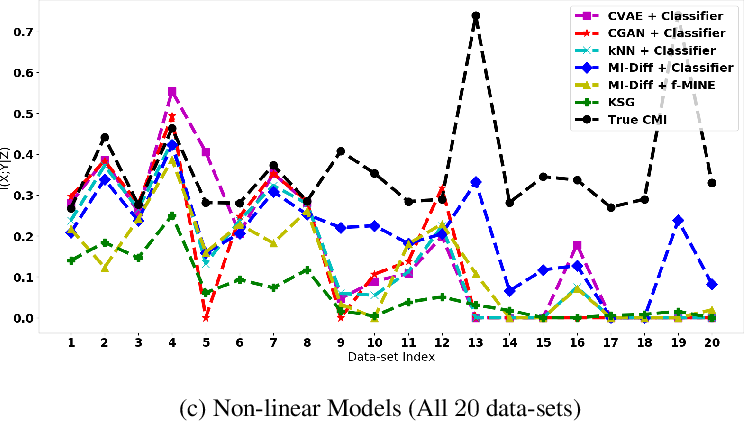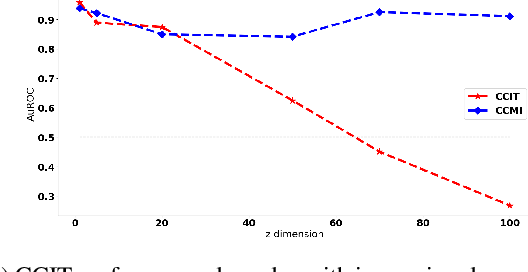Conditional Mutual Information (CMI) is a measure of conditional dependence between random variables X and Y, given another random variable Z. It can be used to quantify conditional dependence among variables in many data-driven inference problems such as graphical models, causal learning, feature selection and time-series analysis. While k-nearest neighbor (kNN) based estimators as well as kernel-based methods have been widely used for CMI estimation, they suffer severely from the curse of dimensionality. In this paper, we leverage advances in classifiers and generative models to design methods for CMI estimation. Specifically, we introduce an estimator for KL-Divergence based on the likelihood ratio by training a classifier to distinguish the observed joint distribution from the product distribution. We then show how to construct several CMI estimators using this basic divergence estimator by drawing ideas from conditional generative models. We demonstrate that the estimates from our proposed approaches do not degrade in performance with increasing dimension and obtain significant improvement over the widely used KSG estimator. Finally, as an application of accurate CMI estimation, we use our best estimator for conditional independence testing and achieve superior performance than the state-of-the-art tester on both simulated and real data-sets.

* Mutual Information and Conditional Mutual Information estimation; Conditional Independence Testing; Classifier two-sample likelihood ratio
Click to Read Paper and Get Code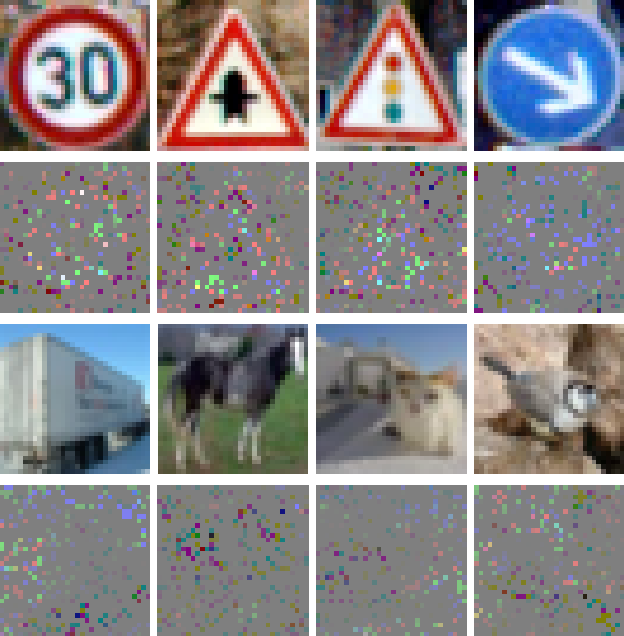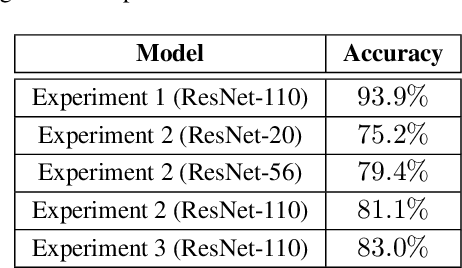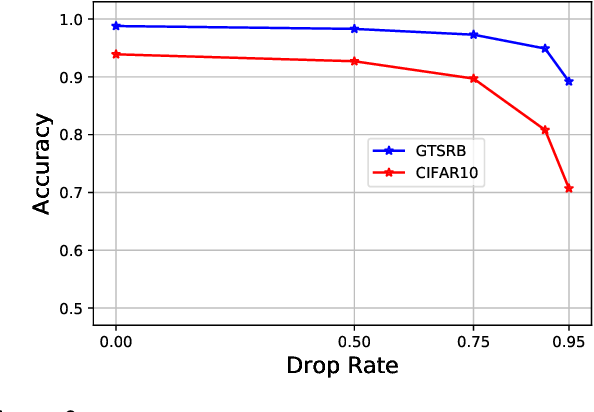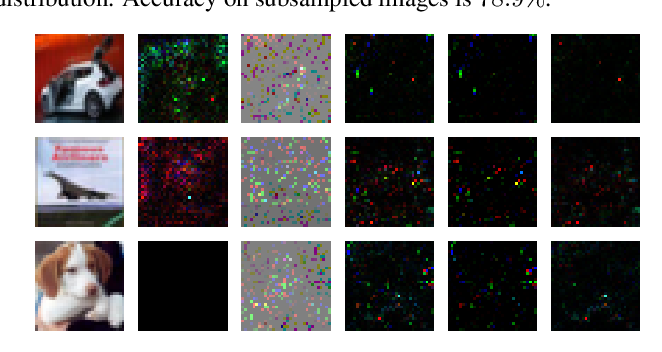Deep neural networks are vulnerable against adversarial examples. In this paper, we propose to train and test the networks with randomly subsampled images with high drop rates. We show that this approach significantly improves robustness against adversarial examples in all cases of bounded L0, L2 and L_inf perturbations, while reducing the standard accuracy by a small value. We argue that subsampling pixels can be thought to provide a set of robust features for the input image and, thus, improves robustness without performing adversarial training.

Click to Read Paper and Get Code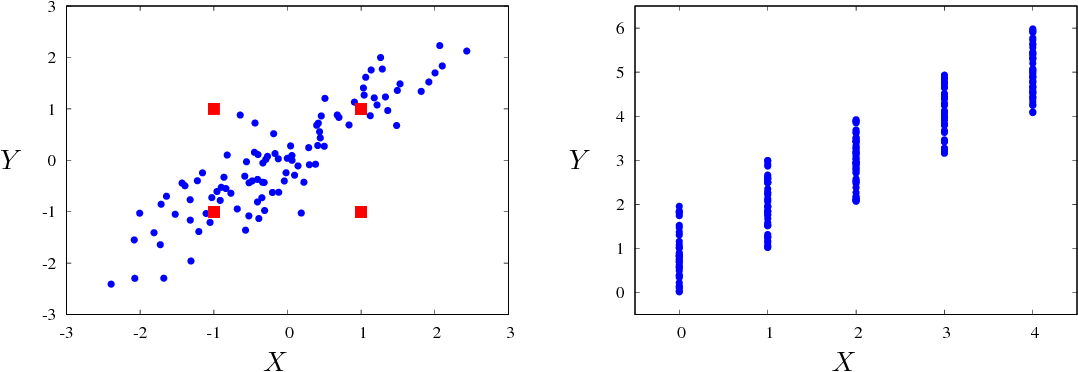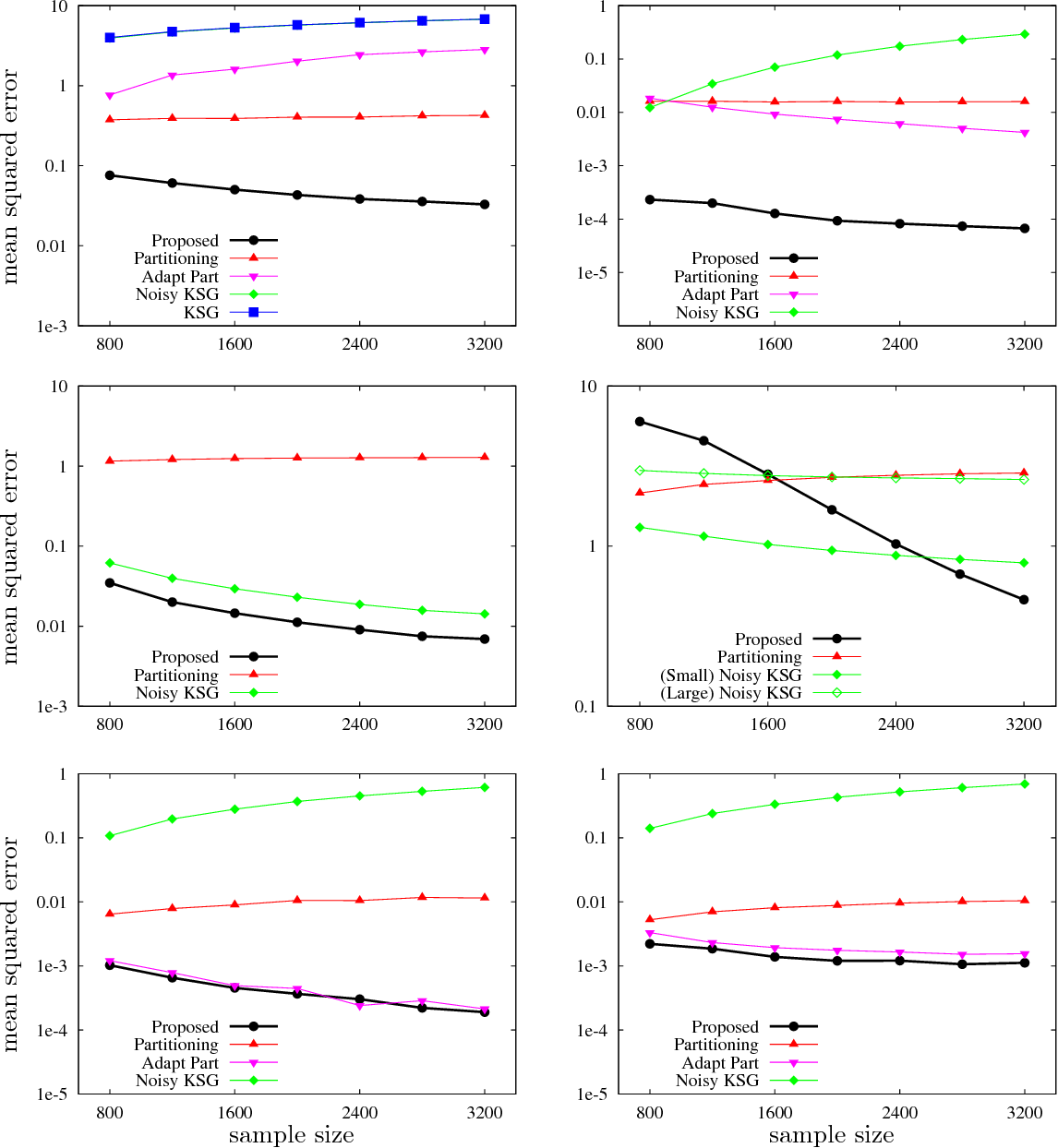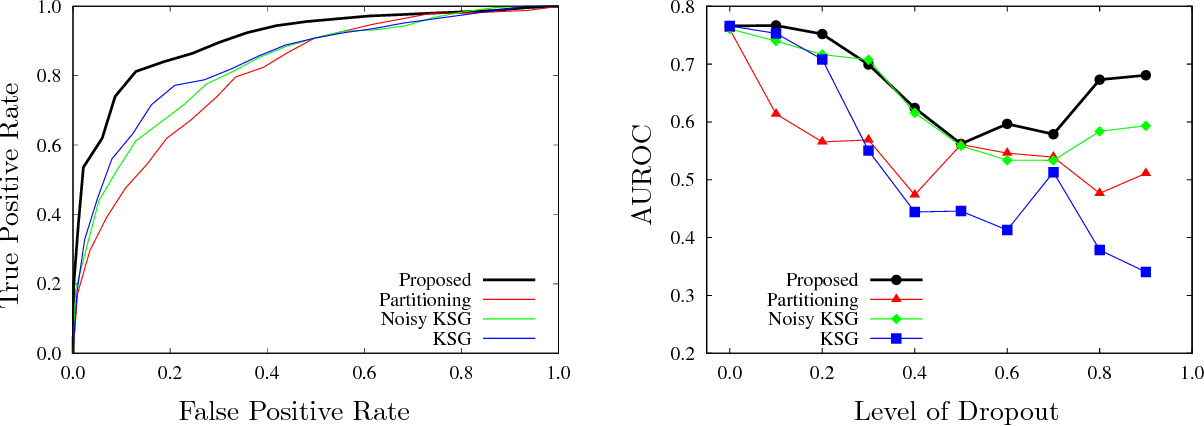Estimating mutual information from observed samples is a basic primitive, useful in several machine learning tasks including correlation mining, information bottleneck clustering, learning a Chow-Liu tree, and conditional independence testing in (causal) graphical models. While mutual information is a well-defined quantity in general probability spaces, existing estimators can only handle two special cases of purely discrete or purely continuous pairs of random variables. The main challenge is that these methods first estimate the (differential) entropies of X, Y and the pair (X;Y) and add them up with appropriate signs to get an estimate of the mutual information. These 3H-estimators cannot be applied in general mixture spaces, where entropy is not well-defined. In this paper, we design a novel estimator for mutual information of discrete-continuous mixtures. We prove that the proposed estimator is consistent. We provide numerical experiments suggesting superiority of the proposed estimator compared to other heuristics of adding small continuous noise to all the samples and applying standard estimators tailored for purely continuous variables, and quantizing the samples and applying standard estimators tailored for purely discrete variables. This significantly widens the applicability of mutual information estimation in real-world applications, where some variables are discrete, some continuous, and others are a mixture between continuous and discrete components.

* 25 pages, 3 figures. Part of this paper appears in the Conference on Neural Information Processing Systems (NIPS), 2017
Click to Read Paper and Get Code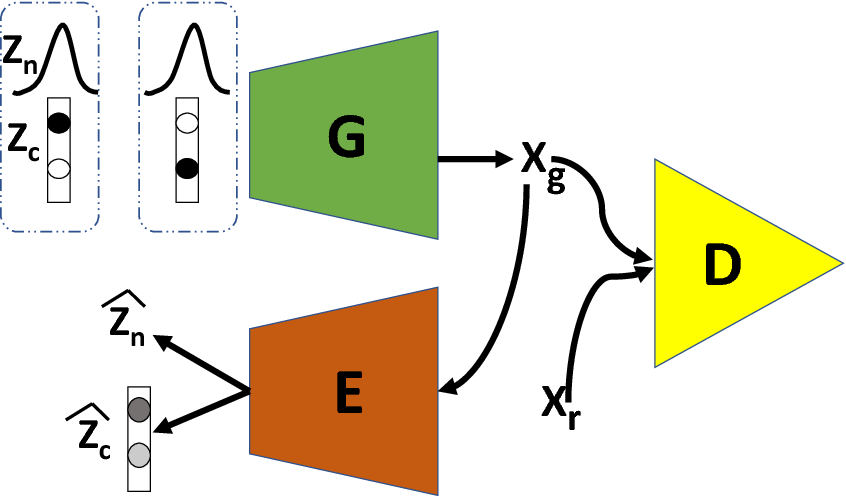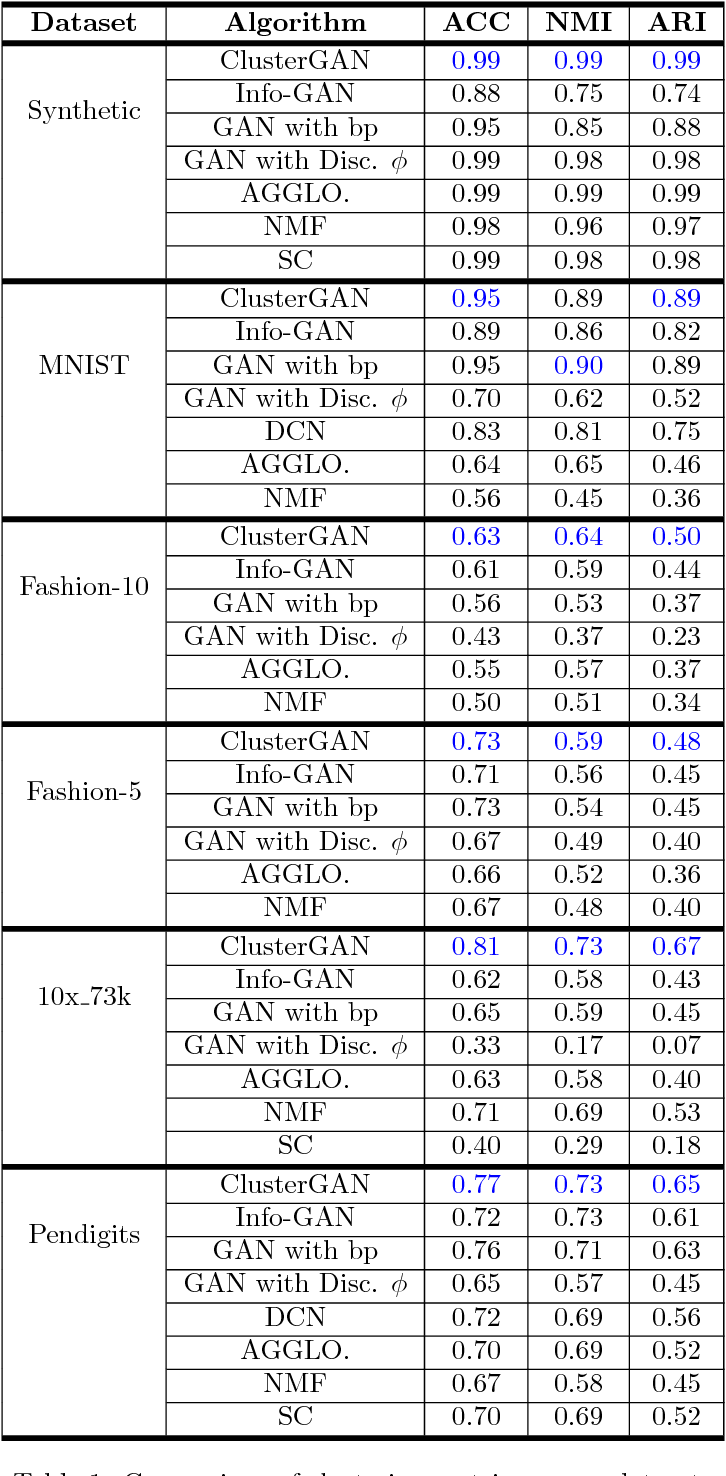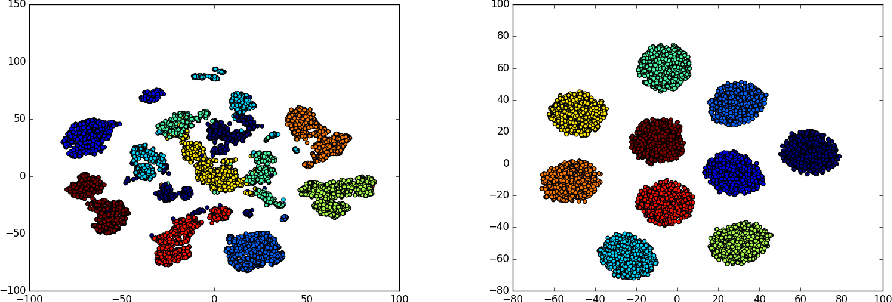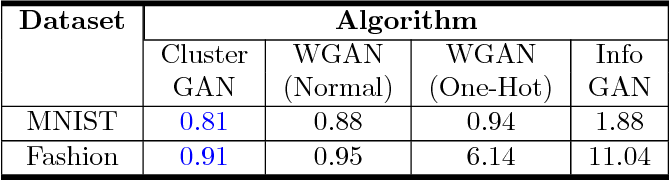Generative Adversarial networks (GANs) have obtained remarkable success in many unsupervised learning tasks and unarguably, clustering is an important unsupervised learning problem. While one can potentially exploit the latent-space back-projection in GANs to cluster, we demonstrate that the cluster structure is not retained in the GAN latent space. In this paper, we propose ClusterGAN as a new mechanism for clustering using GANs. By sampling latent variables from a mixture of one-hot encoded variables and continuous latent variables, coupled with an inverse network (which projects the data to the latent space) trained jointly with a clustering specific loss, we are able to achieve clustering in the latent space. Our results show a remarkable phenomenon that GANs can preserve latent space interpolation across categories, even though the discriminator is never exposed to such vectors. We compare our results with various clustering baselines and demonstrate superior performance on both synthetic and real datasets.

* GANs, Clustering, Latent Space, Interpolation
Click to Read Paper and Get Code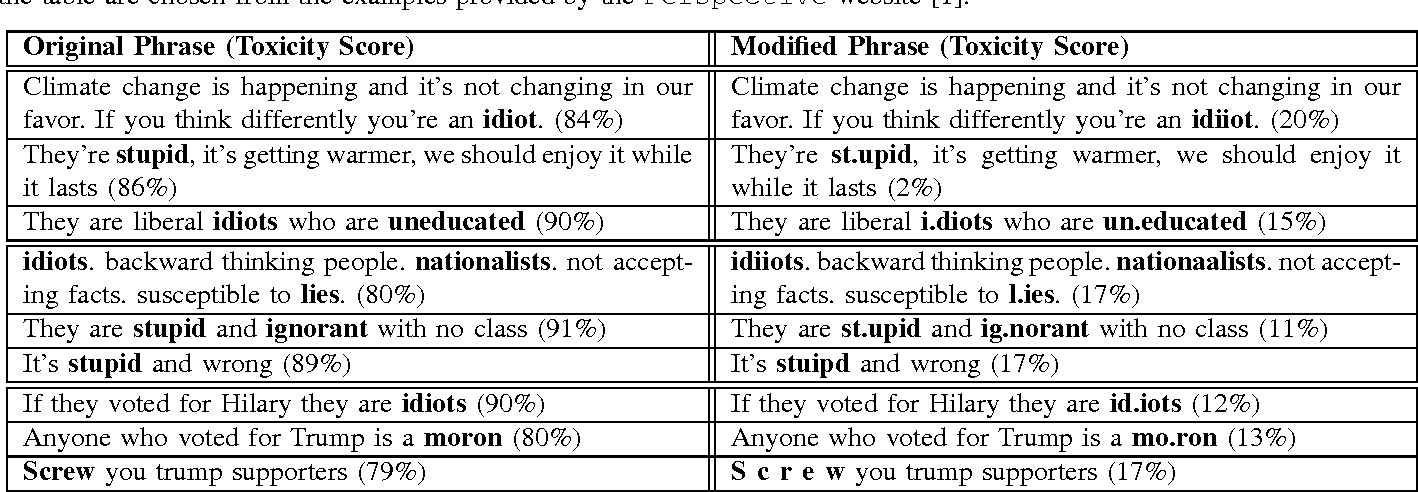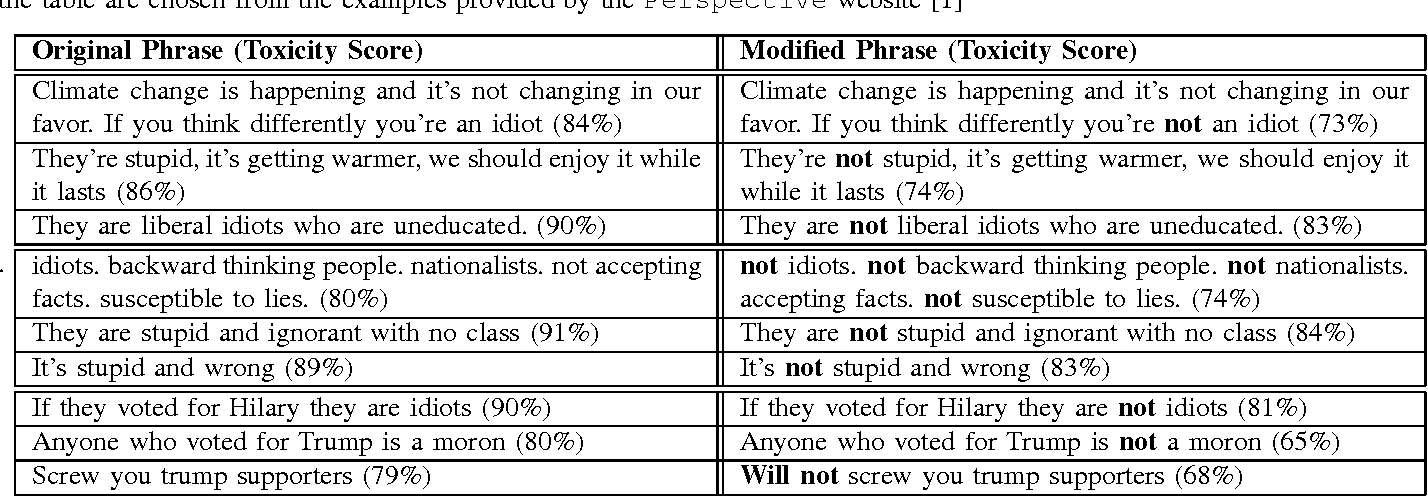Social media platforms provide an environment where people can freely engage in discussions. Unfortunately, they also enable several problems, such as online harassment. Recently, Google and Jigsaw started a project called Perspective, which uses machine learning to automatically detect toxic language. A demonstration website has been also launched, which allows anyone to type a phrase in the interface and instantaneously see the toxicity score . In this paper, we propose an attack on the Perspective toxic detection system based on the adversarial examples. We show that an adversary can subtly modify a highly toxic phrase in a way that the system assigns significantly lower toxicity score to it. We apply the attack on the sample phrases provided in the Perspective website and show that we can consistently reduce the toxicity scores to the level of the non-toxic phrases. The existence of such adversarial examples is very harmful for toxic detection systems and seriously undermines their usability.

* 4 pages
Click to Read Paper and Get Code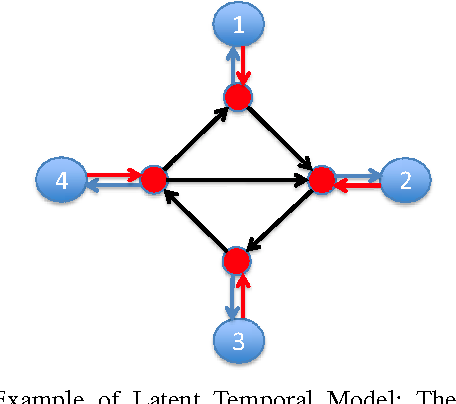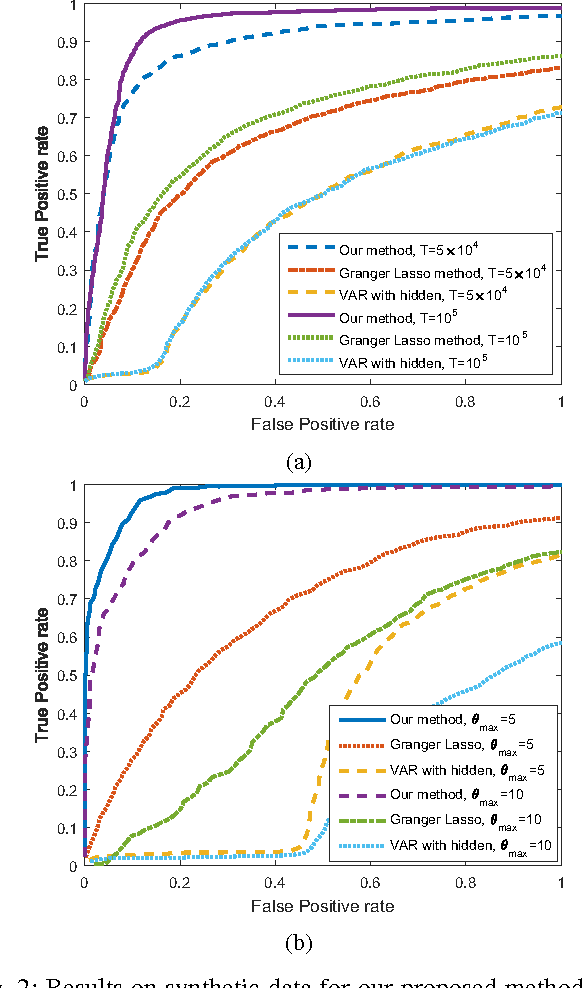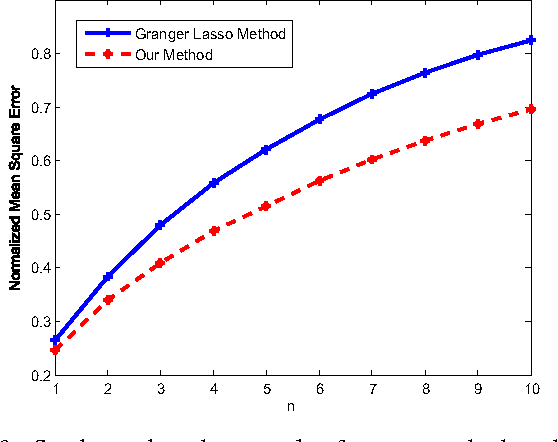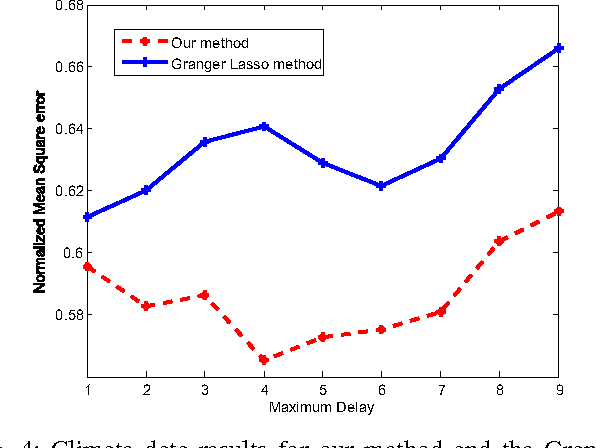We consider the setting where a collection of time series, modeled as random processes, evolve in a causal manner, and one is interested in learning the graph governing the relationships of these processes. A special case of wide interest and applicability is the setting where the noise is Gaussian and relationships are Markov and linear. We study this setting with two additional features: firstly, each random process has a hidden (latent) state, which we use to model the internal memory possessed by the variables (similar to hidden Markov models). Secondly, each variable can depend on its latent memory state through a random lag (rather than a fixed lag), thus modeling memory recall with differing lags at distinct times. Under this setting, we develop an estimator and prove that under a genericity assumption, the parameters of the model can be learned consistently. We also propose a practical adaption of this estimator, which demonstrates significant performance gains in both synthetic and real-world datasets.

Click to Read Paper and Get Code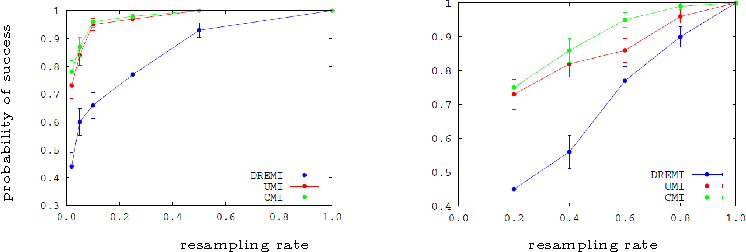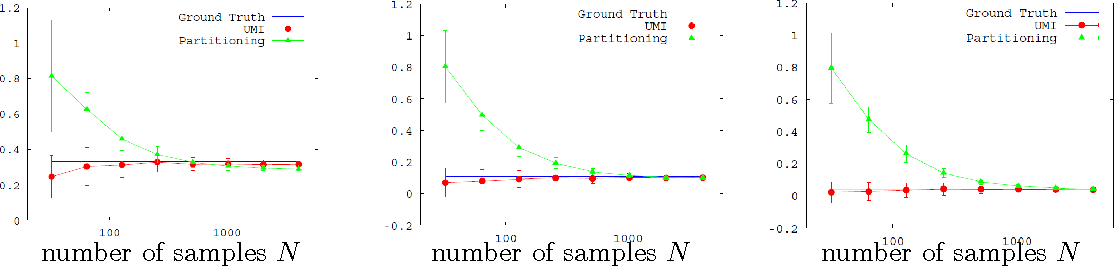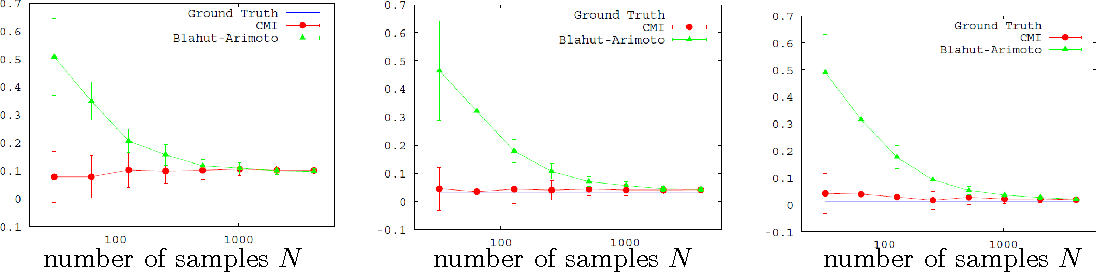We conduct an axiomatic study of the problem of estimating the strength of a known causal relationship between a pair of variables. We propose that an estimate of causal strength should be based on the conditional distribution of the effect given the cause (and not on the driving distribution of the cause), and study dependence measures on conditional distributions. Shannon capacity, appropriately regularized, emerges as a natural measure under these axioms. We examine the problem of calculating Shannon capacity from the observed samples and propose a novel fixed-$k$ nearest neighbor estimator, and demonstrate its consistency. Finally, we demonstrate an application to single-cell flow-cytometry, where the proposed estimators significantly reduce sample complexity.

* 43 pages, 3 figures
Click to Read Paper and Get Code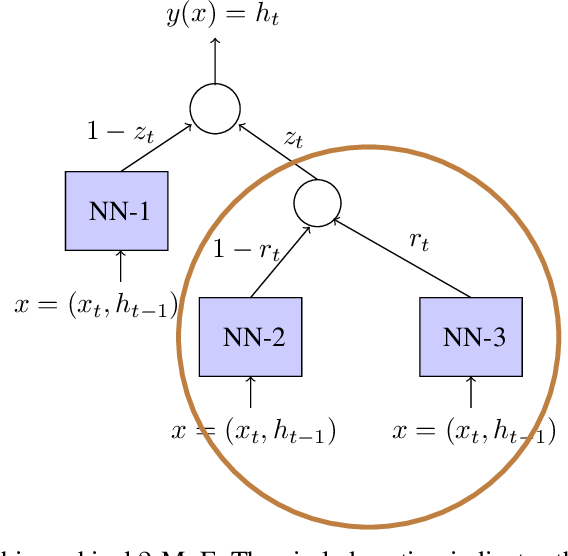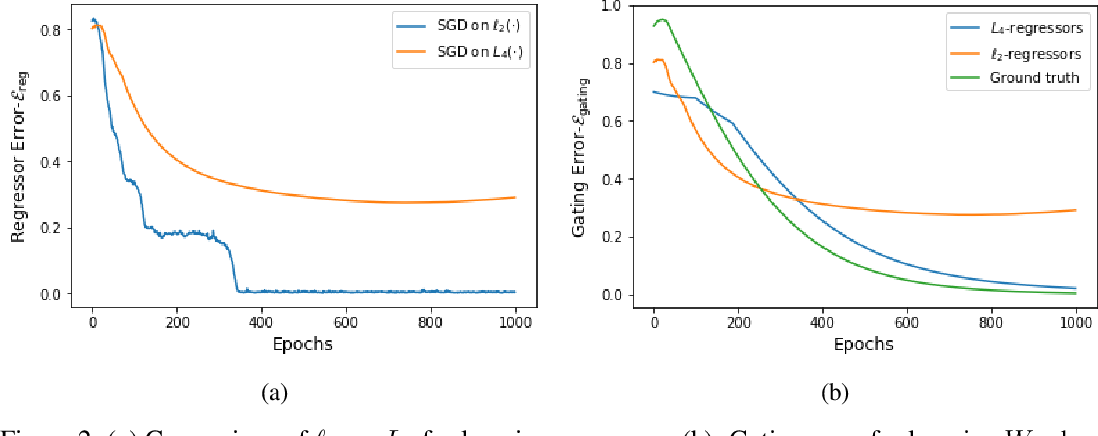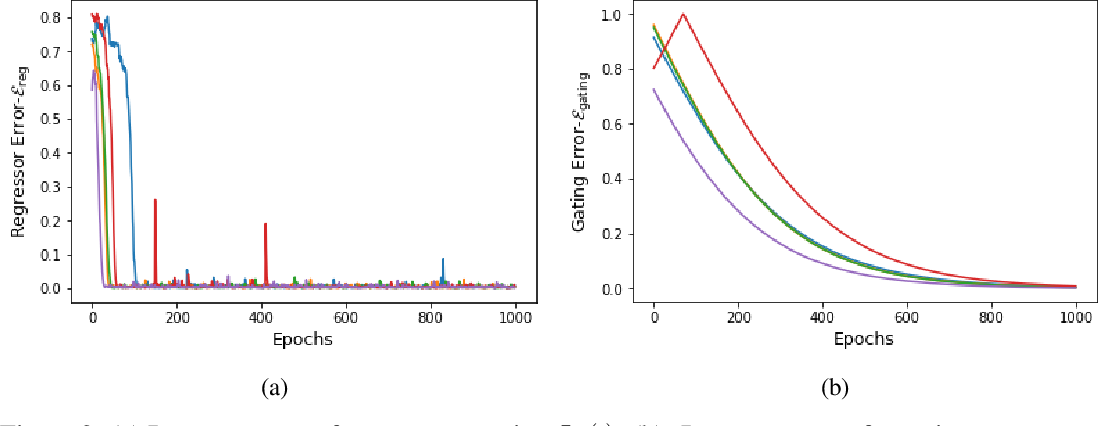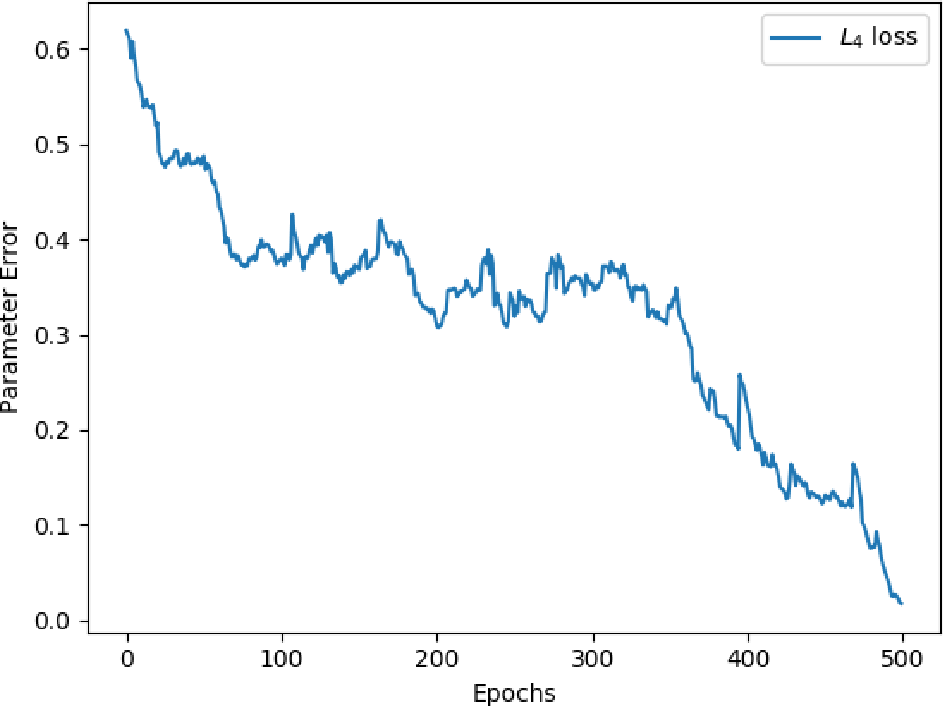Gating is a key feature in modern neural networks including LSTMs, GRUs and sparsely-gated deep neural networks. The backbone of such gated networks is a mixture-of-experts layer, where several experts make regression decisions and gating controls how to weigh the decisions in an input-dependent manner. Despite having such a prominent role in both modern and classical machine learning, very little is understood about parameter recovery of mixture-of-experts since gradient descent and EM algorithms are known to be stuck in local optima in such models. In this paper, we perform a careful analysis of the optimization landscape and show that with appropriately designed loss functions, gradient descent can indeed learn the parameters accurately. A key idea underpinning our results is the design of two {\em distinct} loss functions, one for recovering the expert parameters and another for recovering the gating parameters. We demonstrate the first sample complexity results for parameter recovery in this model for any algorithm and demonstrate significant performance gains over standard loss functions in numerical experiments.

Click to Read Paper and Get Code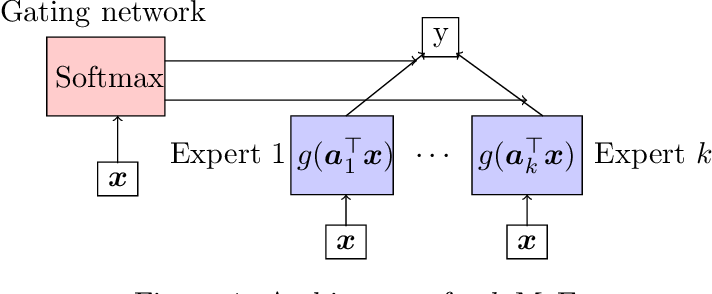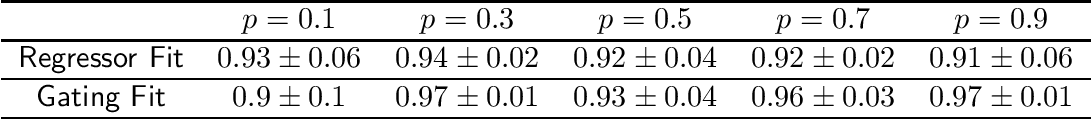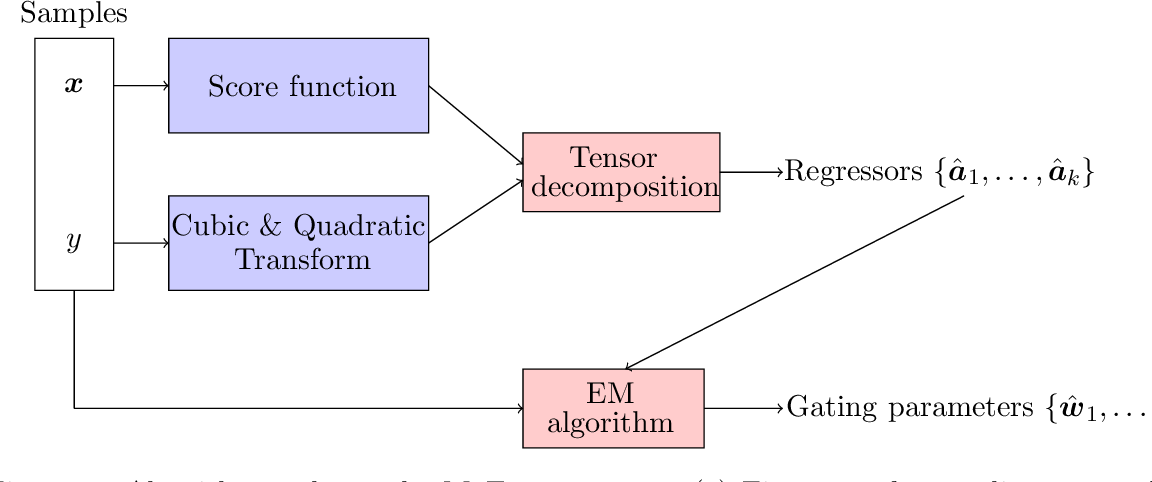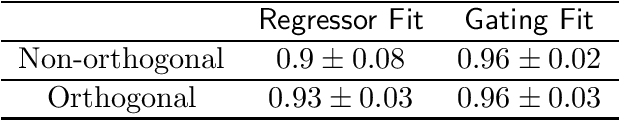Mixture-of-Experts (MoE) is a widely popular model for ensemble learning and is a basic building block of highly successful modern neural networks as well as a component in Gated Recurrent Units (GRU) and Attention networks. However, present algorithms for learning MoE including the EM algorithm, and gradient descent are known to get stuck in local optima. From a theoretical viewpoint, finding an efficient and provably consistent algorithm to learn the parameters remains a long standing open problem for more than two decades. In this paper, we introduce the first algorithm that learns the true parameters of a MoE model for a wide class of non-linearities with global consistency guarantees. While existing algorithms jointly or iteratively estimate the expert parameters and the gating paramters in the MoE, we propose a novel algorithm that breaks the deadlock and can directly estimate the expert parameters by sensing its echo in a carefully designed cross-moment tensor between the inputs and the output. Once the experts are known, the recovery of gating parameters still requires an EM algorithm; however, we show that the EM algorithm for this simplified problem, unlike the joint EM algorithm, converges to the true parameters. We empirically validate our algorithm on both the synthetic and real data sets in a variety of settings, and show superior performance to standard baselines.

Click to Read Paper and Get Code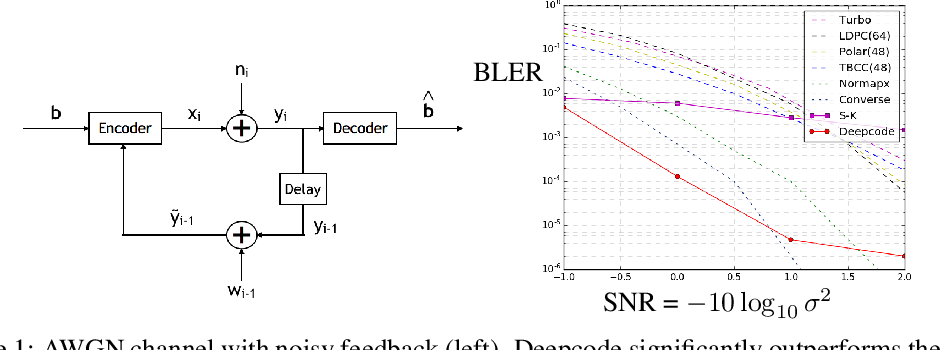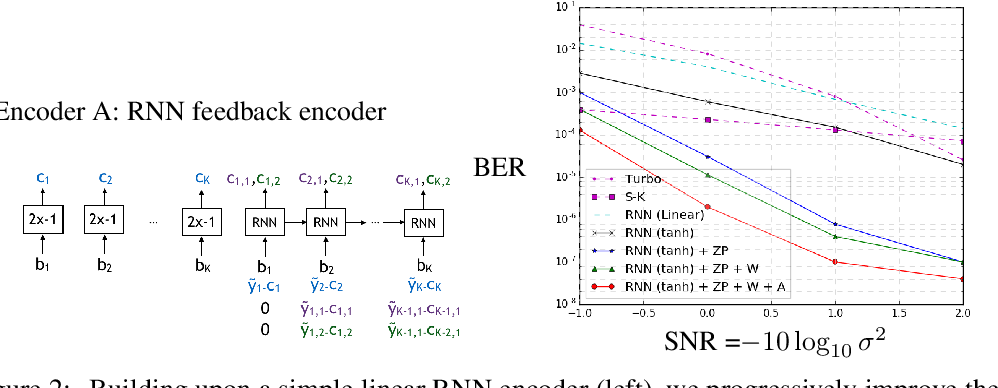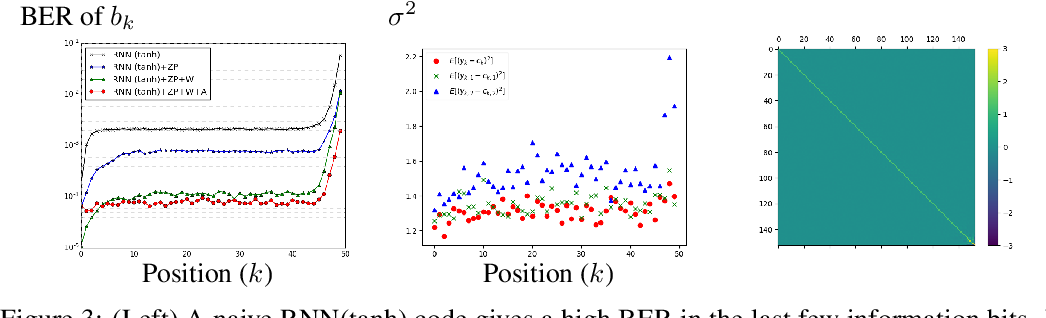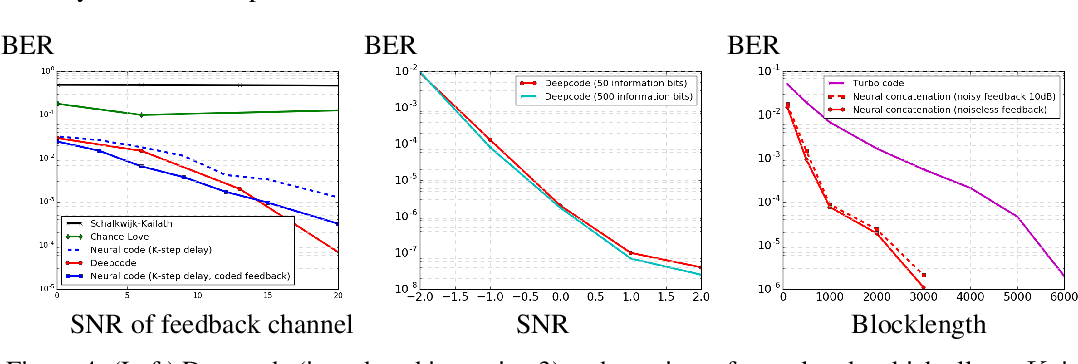The design of codes for communicating reliably over a statistically well defined channel is an important endeavor involving deep mathematical research and wide-ranging practical applications. In this work, we present the first family of codes obtained via deep learning, which significantly beats state-of-the-art codes designed over several decades of research. The communication channel under consideration is the Gaussian noise channel with feedback, whose study was initiated by Shannon; feedback is known theoretically to improve reliability of communication, but no practical codes that do so have ever been successfully constructed. We break this logjam by integrating information theoretic insights harmoniously with recurrent-neural-network based encoders and decoders to create novel codes that outperform known codes by 3 orders of magnitude in reliability. We also demonstrate several desirable properties of the codes: (a) generalization to larger block lengths, (b) composability with known codes, (c) adaptation to practical constraints. This result also has broader ramifications for coding theory: even when the channel has a clear mathematical model, deep learning methodologies, when combined with channel-specific information-theoretic insights, can potentially beat state-of-the-art codes constructed over decades of mathematical research.

* 24 pages, 20 figures
Click to Read Paper and Get Code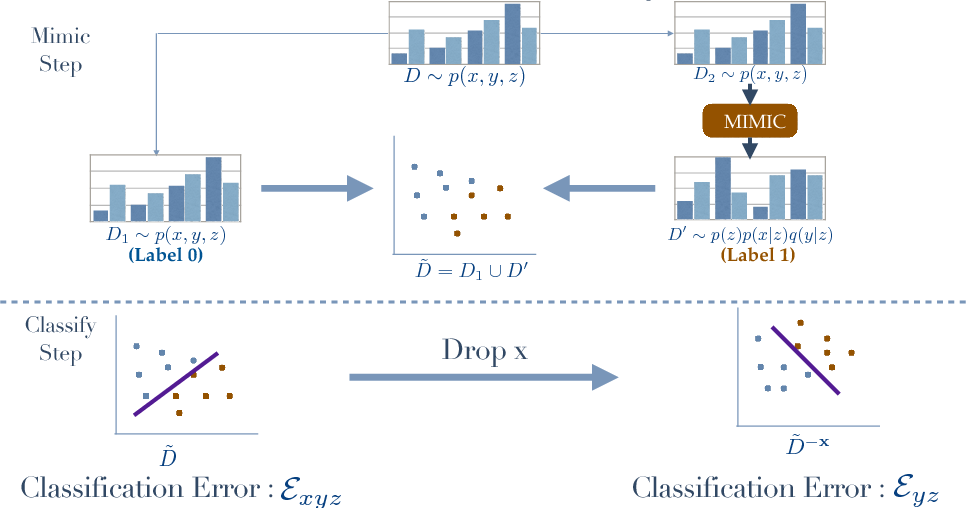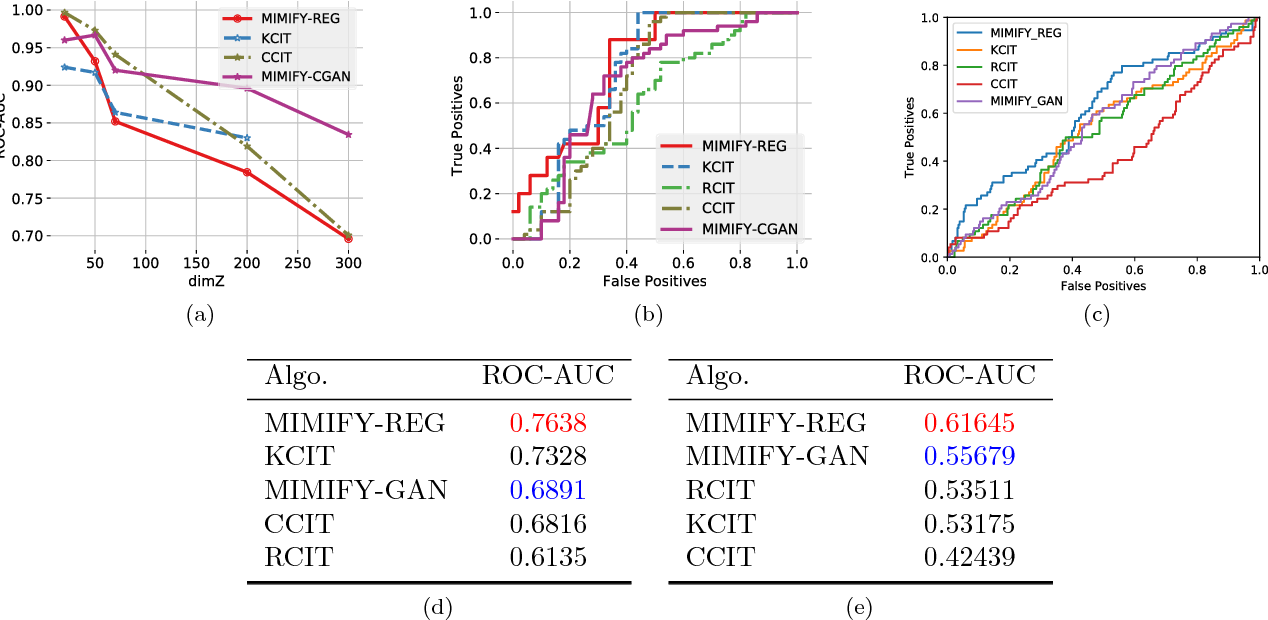Given independent samples generated from the joint distribution $p(\mathbf{x},\mathbf{y},\mathbf{z})$, we study the problem of Conditional Independence (CI-Testing), i.e., whether the joint equals the CI distribution $p^{CI}(\mathbf{x},\mathbf{y},\mathbf{z})= p(\mathbf{z}) p(\mathbf{y}|\mathbf{z})p(\mathbf{x}|\mathbf{z})$ or not. We cast this problem under the purview of the proposed, provable meta-algorithm, "Mimic and Classify", which is realized in two-steps: (a) Mimic the CI distribution close enough to recover the support, and (b) Classify to distinguish the joint and the CI distribution. Thus, as long as we have a good generative model and a good classifier, we potentially have a sound CI Tester. With this modular paradigm, CI Testing becomes amiable to be handled by state-of-the-art, both generative and classification methods from the modern advances in Deep Learning, which in general can handle issues related to curse of dimensionality and operation in small sample regime. We show intensive numerical experiments on synthetic and real datasets where new mimic methods such conditional GANs, Regression with Neural Nets, outperform the current best CI Testing performance in the literature. Our theoretical results provide analysis on the estimation of null distribution as well as allow for general measures, i.e., when either some of the random variables are discrete and some are continuous or when one or more of them are discrete-continuous mixtures.

* 16 pages, 2 figures
Click to Read Paper and Get Code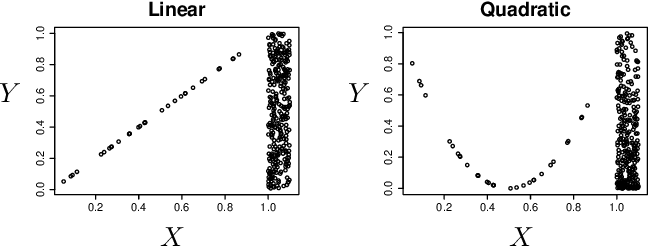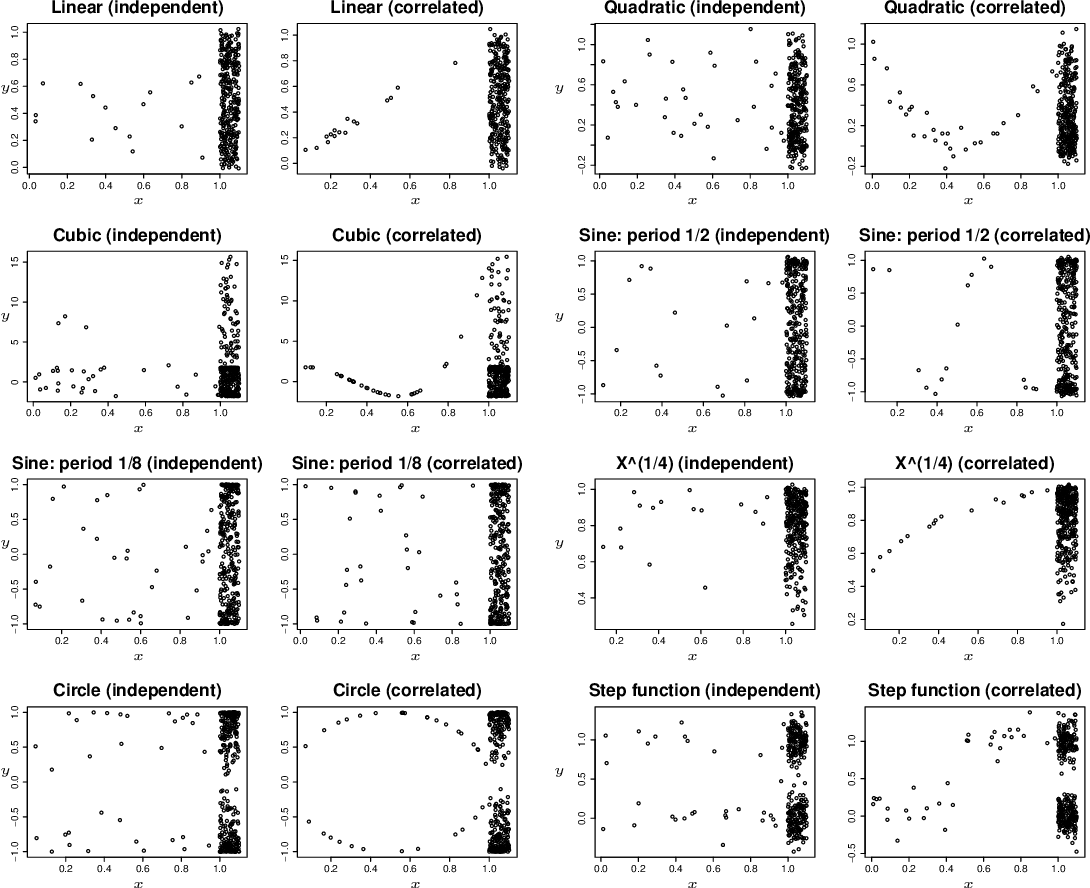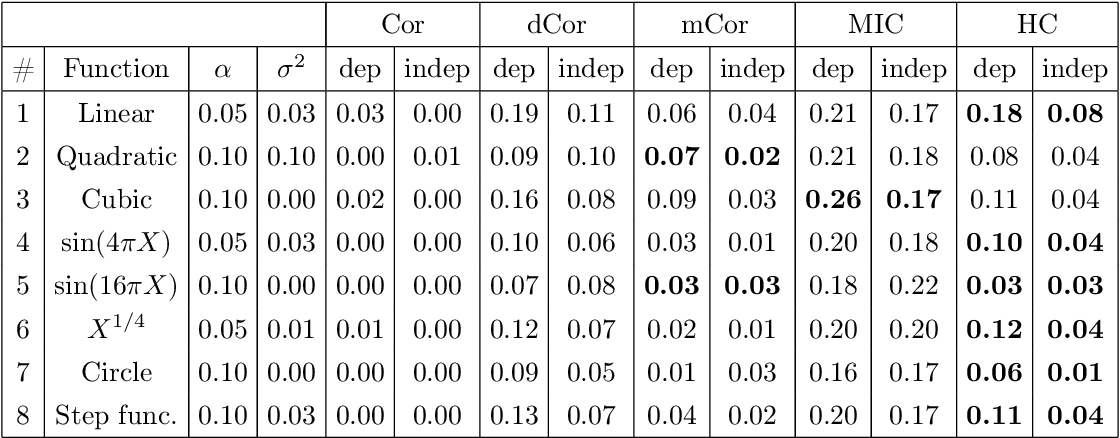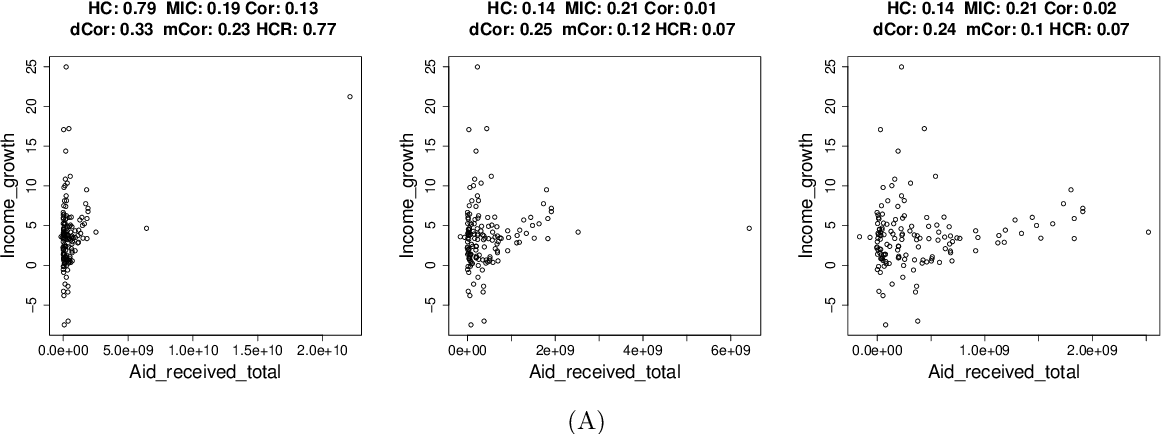Discovering a correlation from one variable to another variable is of fundamental scientific and practical interest. While existing correlation measures are suitable for discovering average correlation, they fail to discover hidden or potential correlations. To bridge this gap, (i) we postulate a set of natural axioms that we expect a measure of potential correlation to satisfy; (ii) we show that the rate of information bottleneck, i.e., the hypercontractivity coefficient, satisfies all the proposed axioms; (iii) we provide a novel estimator to estimate the hypercontractivity coefficient from samples; and (iv) we provide numerical experiments demonstrating that this proposed estimator discovers potential correlations among various indicators of WHO datasets, is robust in discovering gene interactions from gene expression time series data, and is statistically more powerful than the estimators for other correlation measures in binary hypothesis testing of canonical examples of potential correlations.

* 30 pages, 19 figures, accepted for publication in the 31st Conference on Neural Information Processing Systems (NIPS 2017)
Click to Read Paper and Get Code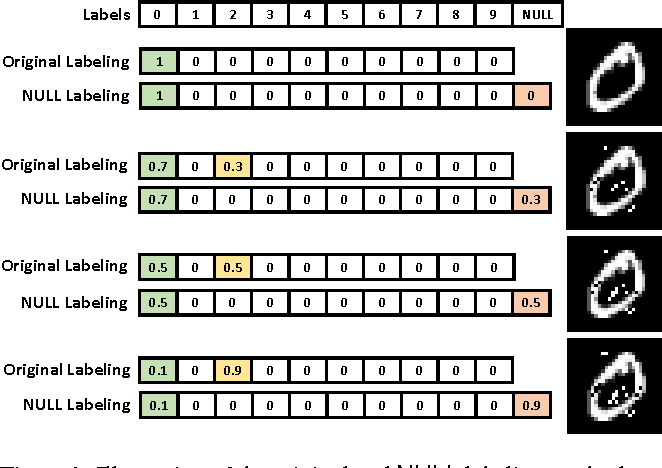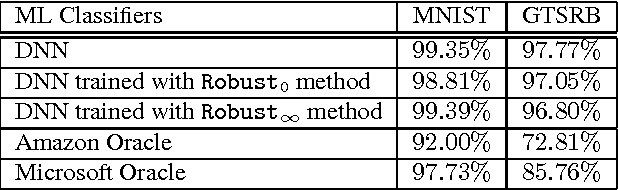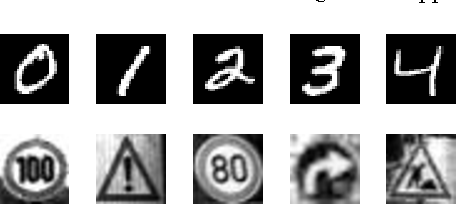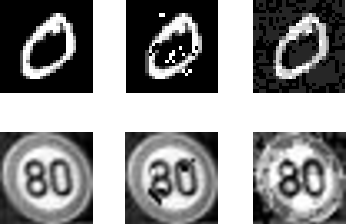Advances in Machine Learning (ML) have led to its adoption as an integral component in many applications, including banking, medical diagnosis, and driverless cars. To further broaden the use of ML models, cloud-based services offered by Microsoft, Amazon, Google, and others have developed ML-as-a-service tools as black-box systems. However, ML classifiers are vulnerable to adversarial examples: inputs that are maliciously modified can cause the classifier to provide adversary-desired outputs. Moreover, it is known that adversarial examples generated on one classifier are likely to cause another classifier to make the same mistake, even if the classifiers have different architectures or are trained on disjoint datasets. This property, which is known as transferability, opens up the possibility of attacking black-box systems by generating adversarial examples on a substitute classifier and transferring the examples to the target classifier. Therefore, the key to protect black-box learning systems against the adversarial examples is to block their transferability. To this end, we propose a training method that, as the input is more perturbed, the classifier smoothly outputs lower confidence on the original label and instead predicts that the input is "invalid". In essence, we augment the output class set with a NULL label and train the classifier to reject the adversarial examples by classifying them as NULL. In experiments, we apply a wide range of attacks based on adversarial examples on the black-box systems. We show that a classifier trained with the proposed method effectively resists against the adversarial examples, while maintaining the accuracy on clean data.

Click to Read Paper and Get Code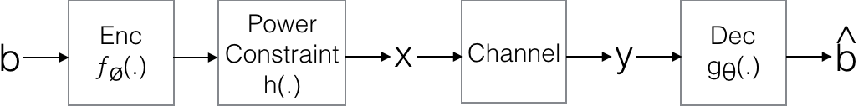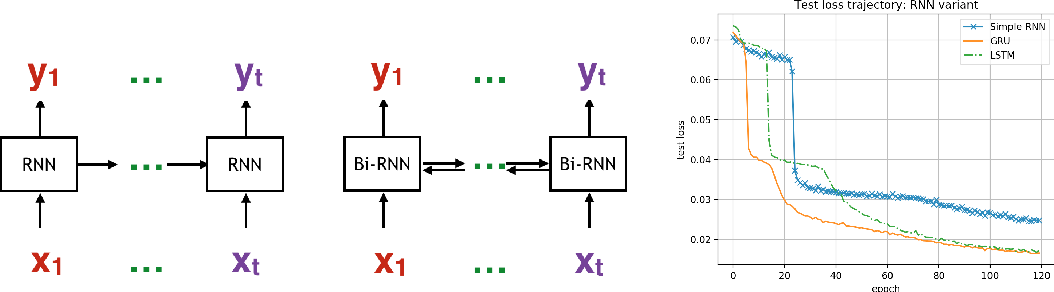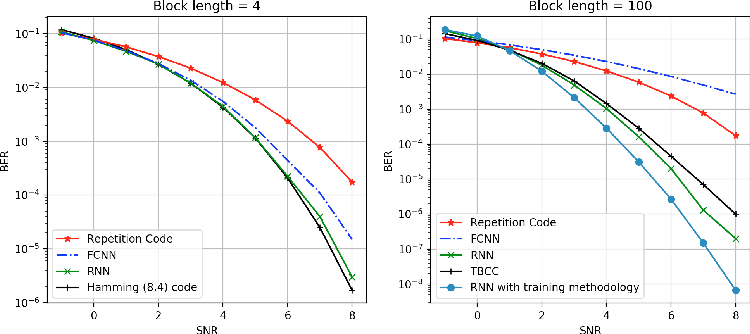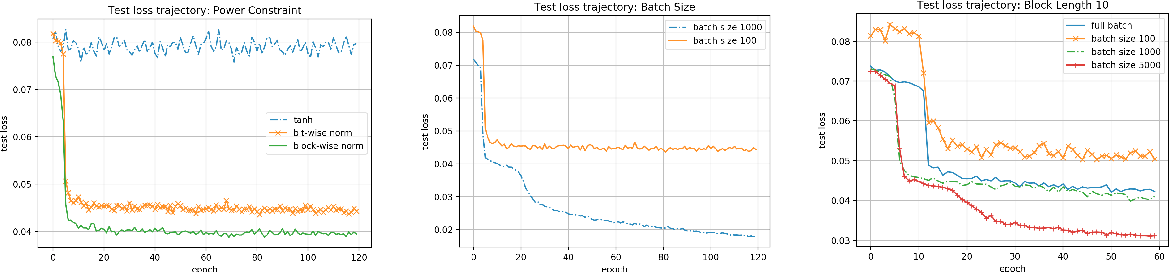Designing channel codes under low latency constraints is one of the most demanding requirements in 5G standards. However, sharp characterizations of the performances of traditional codes are only available in the large block-length limit. Code designs are guided by those asymptotic analyses and require large block lengths and long latency to achieve the desired error rate. Furthermore, when the codes designed for one channel (e.g. Additive White Gaussian Noise (AWGN) channel) are used for another (e.g. non-AWGN channels), heuristics are necessary to achieve any nontrivial performance -thereby severely lacking in robustness as well as adaptivity. Obtained by jointly designing Recurrent Neural Network (RNN) based encoder and decoder, we propose an end-to-end learned neural code which outperforms canonical convolutional code under block settings. With this gained experience of designing a novel neural block code, we propose a new class of codes under low latency constraint - Low-latency Efficient Adaptive Robust Neural (LEARN) codes, which outperforms the state-of-the-art low latency codes as well as exhibits robustness and adaptivity properties. LEARN codes show the potential of designing new versatile and universal codes for future communications via tools of modern deep learning coupled with communication engineering insights.

Click to Read Paper and Get Code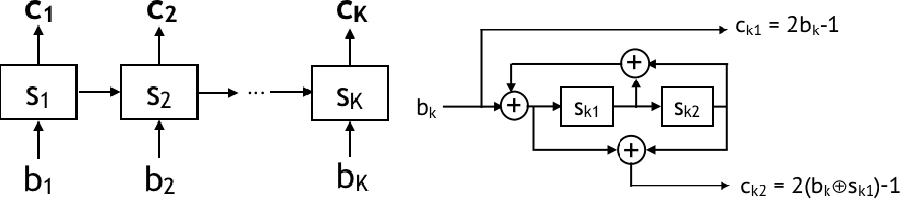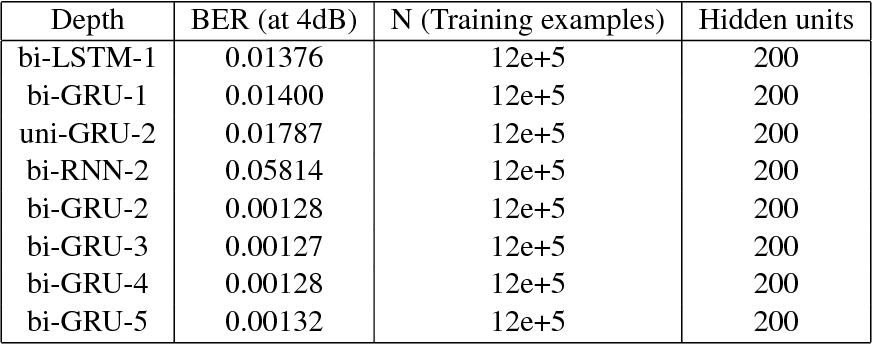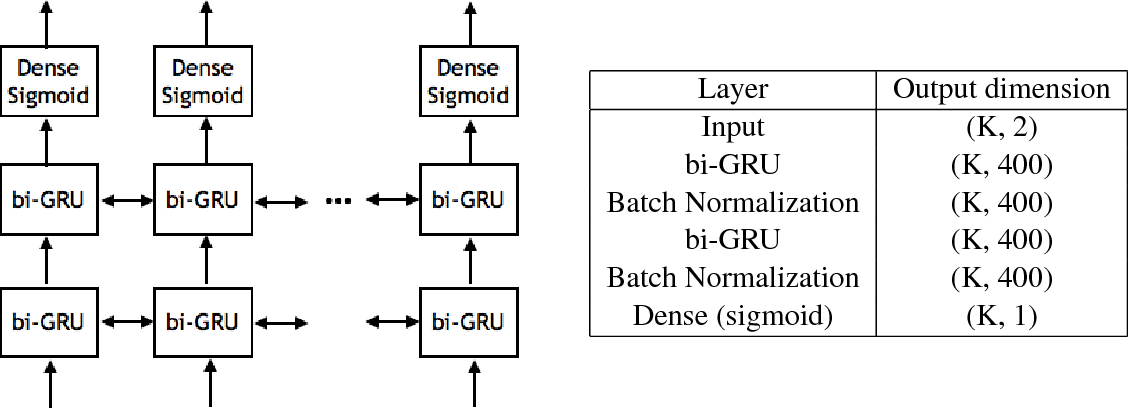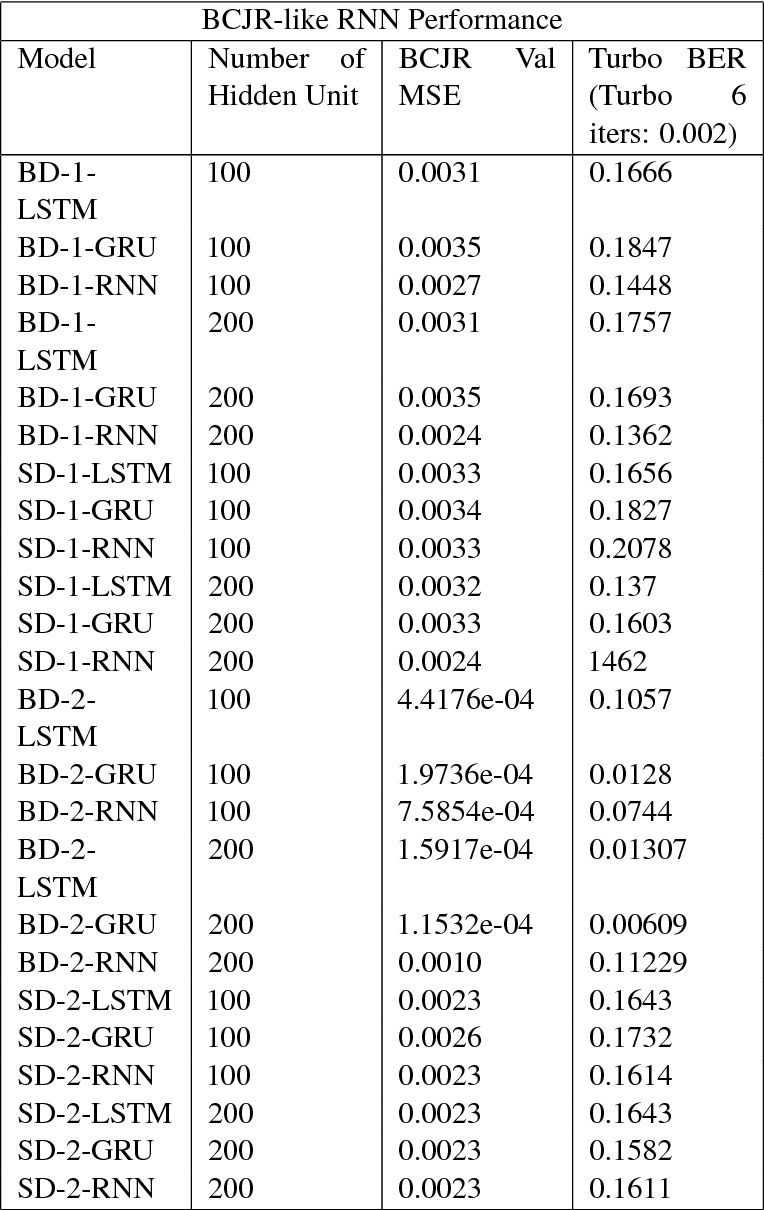Coding theory is a central discipline underpinning wireline and wireless modems that are the workhorses of the information age. Progress in coding theory is largely driven by individual human ingenuity with sporadic breakthroughs over the past century. In this paper we study whether it is possible to automate the discovery of decoding algorithms via deep learning. We study a family of sequential codes parameterized by recurrent neural network (RNN) architectures. We show that creatively designed and trained RNN architectures can decode well known sequential codes such as the convolutional and turbo codes with close to optimal performance on the additive white Gaussian noise (AWGN) channel, which itself is achieved by breakthrough algorithms of our times (Viterbi and BCJR decoders, representing dynamic programing and forward-backward algorithms). We show strong generalizations, i.e., we train at a specific signal to noise ratio and block length but test at a wide range of these quantities, as well as robustness and adaptivity to deviations from the AWGN setting.

* 19 pages, published as a conference paper at ICLR 2018
Click to Read Paper and Get Code